# 诡异秘境：懒惰镇

## 懒惰镇篇（上册）第7章 秋琼，我的名字（3）

[更新时间] 2019-05-15 14:44:17 [字数] 3992

“噼啪……噼啪……”~|\$+=本书%首-发纵-横中文=网=\$@|&

“噼啪……噼啪……”房间很明亮，又很温暖。~|\$+=本书%首-发纵-横中文=网=\$@|&

“那个……我在昏睡的时候，做了个梦，梦见了一个女人。我想不起来她是谁，她就站在我的面前……叫我不要放弃……我不知道她说的放弃是要放弃什么，也不知道她说的那个他到底是谁？”~|\$+=本书%首-发纵-横中文=网=\$@|&

“……嗯……”~|\$+=本书%首-发纵-横中文=网=\$@|&

“对了，老许，我知道自己的名字了。我叫秋琼，那个梦里的女人告诉我的。”~|\$+=本书%首-发纵-横中文=网=\$@|&

“嗯？秋……琼……好名字。”老许念着我的名字，左手已经捂着自己的嘴巴，右手拖着左手的手肘，眉头一直紧锁，眼神更是淡然无光。~|\$+=本书%首-发纵-横中文=网=\$@|&

“你是不是有什么话要对我说呀。看你的表情，感觉我有什么不好的事情。你就直说吧，本小姐大心脏得很。”我试探性地问了一问。~|\$+=本书%首-发纵-横中文=网=\$@|&

“琼姑娘……我……”老许向前走了一步，一副若有所思的样子。~|\$+=本书%首-发纵-横中文=网=\$@|&

“不要叫琼姑娘啦，你可以直接喊我的名字啊，琼也可以，或者小琼，我都可以接受。”~|\$+=本书%首-发纵-横中文=网=\$@|&

“啊呵，小琼姑娘……小琼……我有很多事要跟你说，但是我怕你一时间没有办法理解。”~|\$+=本书%首-发纵-横中文=网=\$@|&

“很多事情要跟我说？大概是什么啊？”~|\$+=本书%首-发纵-横中文=网=\$@|&

“说起来也没什么大事，之后还会有人跟你详细地说，我就来给你打个底。毕竟你初来乍到，对我们这里的环境、条件不大了解。我等一下要说的事，你也不用太惊讶，因为在我们这里，一切都很正常。”~|\$+=本书%首-发纵-横中文=网=\$@|&

“嗯……你就说吧。”我点了点头，示意他可以继续说下去。~|\$+=本书%首-发纵-横中文=网=\$@|&

“小琼，我就打个比方，从简单的跟你说吧。你看这个火，它有什么特别吗？”~|\$+=本书%首-发纵-横中文=网=\$@|&

“嗯……没什么特别的啊。”~|\$+=本书%首-发纵-横中文=网=\$@|&

“好，那我现在让它变小。”~|\$+=本书%首-发纵-横中文=网=\$@|&

“这……是魔法吗？”~|\$+=本书%首-发纵-横中文=网=\$@|&

“不是，这不是魔法。你过来。”~|\$+=本书%首-发纵-横中文=网=\$@|&

“小琼，你过来摸摸它？”~|\$+=本书%首-发纵-横中文=网=\$@|&

“什……什么？摸啥？摸这个火？”~|\$+=本书%首-发纵-横中文=网=\$@|&

“是啊。快过来。”~|\$+=本书%首-发纵-横中文=网=\$@|&

“你疯了吧。玩啥不好，玩火？”~|\$+=本书%首-发纵-横中文=网=\$@|&

“我绝对没疯。你快过来，很好玩的。”~|\$+=本书%首-发纵-横中文=网=\$@|&

“你到底要搞什么？”~|\$+=本书%首-发纵-横中文=网=\$@|&

——我的手在火焰里居然一点事都没有。它的确包裹着我的手臂，可我并没有因此而烧伤。~|\$+=本书%首-发纵-横中文=网=\$@|&

“你心里肯定觉得很奇怪吧。我跟你说噢，这是智械。”~|\$+=本书%首-发纵-横中文=网=\$@|&

“智……什么？！”~|\$+=本书%首-发纵-横中文=网=\$@|&

“智械，全名叫智能机械。你看这底下的干柴，它看起像真的，但它其实是一个机械装置，叫智能燃火器，能根据人的意念调节火焰的大小和温度。当然了，它不会伤到你，是因为它的火焰同样也是智能的。你手一伸进去，火焰的轨迹就会自动调整，躲避与人接触，十分安全。”~|\$+=本书%首-发纵-横中文=网=\$@|&

“这……怎么会呢？这世上已经有这么先进的技术了？”~|\$+=本书%首-发纵-横中文=网=\$@|&

“哈哈，别的地方我不敢说，但这里还真有。”~|\$+=本书%首-发纵-横中文=网=\$@|&

“……”我一时愣得说不出话来了。~|\$+=本书%首-发纵-横中文=网=\$@|&

“那我再举个例子吧。就你刚刚吃的饭也不是人煮的，我们把食材放好，后台的机械会根据今天多少人要吃饭，做多少饭出来。而就在我们带你来到这里的时候，机械算出今天你要吃饭，所以自动调整了，把你的那一份饭也做出来了。要不然，我们总有一个人没饭吃。”~|\$+=本书%首-发纵-横中文=网=\$@|&

“……”~|\$+=本书%首-发纵-横中文=网=\$@|&

“我……我觉得不可能啊！”~|\$+=本书%首-发纵-横中文=网=\$@|&

“哈哈，没事，一开始确实挺难接受的。我们懒惰镇最不缺的就是智能机械。这里的每一样东西，肉眼所见没有一件不是机械装置，有的单纯只是机械，有的却是智能的。单纯机械的你看这个桌子，它上面娱乐模式、学习模式等都需要你动手操作，它不能读取你的需求进行自动调整。而智能机械的话，你往后面的床看一下，你对着床喊一声。”~|\$+=本书%首-发纵-横中文=网=\$@|&

“……喊什么？”~|\$+=本书%首-发纵-横中文=网=\$@|&

“你就……喊一句……沙发吧。”~|\$+=本书%首-发纵-横中文=网=\$@|&

“沙发，沙发！”~|\$+=本书%首-发纵-横中文=网=\$@|&

“吱--吱--”那张小床竟真的开始自动折叠起来，变成了一个沙发。~|\$+=本书%首-发纵-横中文=网=\$@|&

“你看……它就变成一个沙发了吧。你在看看这个水壶，现在没有水吧。我把它放在这配套的装置上，你再喊一句试试？”~|\$+=本书%首-发纵-横中文=网=\$@|&

“热水！哇，真的出热水了。”~|\$+=本书%首-发纵-横中文=网=\$@|&

“聪明。你再喊一句冷水试试？”~|\$+=本书%首-发纵-横中文=网=\$@|&

“冰！我的天，这！”~|\$+=本书%首-发纵-横中文=网=\$@|&

“你看吧……这热腾腾的水结冰了。”~|\$+=本书%首-发纵-横中文=网=\$@|&

“……这世上真有这么先进的东西？不会是有人在背后操控的吧。”~|\$+=本书%首-发纵-横中文=网=\$@|&

“当然有操控的人……那就是你。刚刚我已经正式授权你成为这个房间的主人了，这里面的机械别人没有办法操作，你可以根据自己的需要，任意使唤它们。这是我们总结的一份说明书，有什么不懂的地方，可以看看。等它适应了你的想法和需求，你就不需要命令它们，它们会自动跟上你节奏。”~|\$+=本书%首-发纵-横中文=网=\$@|&

“你们是怎么办到的？一个全机械的地方？”~|\$+=本书%首-发纵-横中文=网=\$@|&

“嗯，我现在告诉你怎么办到的还有点早，等会儿……会有人告诉你的。不过至于怎么会存在嘛，它真的存在，而且就在你所处的地方。”老许很自豪地说道。~|\$+=本书%首-发纵-横中文=网=\$@|&

“我……”~|\$+=本书%首-发纵-横中文=网=\$@|&

“没事，你会慢慢适应的。”~|\$+=本书%首-发纵-横中文=网=\$@|&

“这些天碰到的事，真的让我的头有点大。”~|\$+=本书%首-发纵-横中文=网=\$@|&

“小琼，你有什么想问的吗？我都可以尽力帮你解答哟！”~|\$+=本书%首-发纵-横中文=网=\$@|&

“啊，我头大！欸……那我大胆地猜一下，老许你忽然之间变这么年轻，也肯定跟智能机械有关咯？”~|\$+=本书%首-发纵-横中文=网=\$@|&

“哈哈，你挺聪明的啊！你还记得我爬向的那张床吗？那可不是一张普通的床，它是一台‘疗生机’。”~|\$+=本书%首-发纵-横中文=网=\$@|&

“疗生机又是什么鬼？”~|\$+=本书%首-发纵-横中文=网=\$@|&

“它是一种能促进人体细胞再生和加快代谢循环的机械，只要在上面躺上一定时日，人也就恢复年轻了。”~|\$+=本书%首-发纵-横中文=网=\$@|&

“可我看到你的时候，你怎么是个大叔模样呢？”~|\$+=本书%首-发纵-横中文=网=\$@|&

“唉，这是疗生机的副作用，这个疗生机如果你不定时使用的话，你的身体反而会快速地衰老，甚至有致命的危险，这就是‘那家伙’困住我们的方式。但是，我作为镇上唯一出城运送物资的人，每个月要出去一趟要很久，所以我的副作用要比其他人小一些，衰老得慢点，你也就看得到我中年的模样。”~|\$+=本书%首-发纵-横中文=网=\$@|&

“那家伙是？” ~|\$+=本书%首-发纵-横中文=网=\$@|&

“噗……山山，哈哈！”~|\$+=本书%首-发纵-横中文=网=\$@|&

“啧，别笑！嘘！”~|\$+=本书%首-发纵-横中文=网=\$@|&

“山山~原来你在这啊!”那个小女生挽着老许的胳膊，娇羞地说道。~|\$+=本书%首-发纵-横中文=网=\$@|&

“噢~这是……人工智能吗？”我问。~|\$+=本书%首-发纵-横中文=网=\$@|&

“呵呵，你别看我们这里的机械是智能的，就把我们和‘人工智能’那种机械人联系在一起。外面有个地方就全是人工智能机械人，相信我，你绝对不想和它们待上一分一秒。” 提起“人工智能”四个字，老许的脸上明显变得气愤和厌恶。~|\$+=本书%首-发纵-横中文=网=\$@|&

“啊？哪里？” 我追问道。~|\$+=本书%首-发纵-横中文=网=\$@|&

“山山~你怎么不理我！”那个小女生见老许没有搭理她，嘟起了嘴。~|\$+=本书%首-发纵-横中文=网=\$@|&

“好啦，好啦。我不正忙嘛！”~|\$+=本书%首-发纵-横中文=网=\$@|&

“山山~这是谁啊？我怎么没看到这有个人？”她立即躲在了老许身后，只露出半边脸偷偷看我。~|\$+=本书%首-发纵-横中文=网=\$@|&

“这就是昨晚我想你提过的呀……我们的救星。”~|\$+=本书%首-发纵-横中文=网=\$@|&

“噢~原来你就是大救星！你好啊，我叫姜蔓。”~|\$+=本书%首-发纵-横中文=网=\$@|&

“你好，秋琼，我的名字。”~|\$+=本书%首-发纵-横中文=网=\$@|&

“什么？等等！救星？！”~|\$+=本书%首-发纵-横中文=网=\$@|&

#### 作者有话说：

[+展开]
(快捷键：←)上一章 回目录(快捷键：回车) 下一章(快捷键：→)

### 发表评论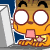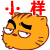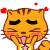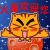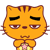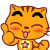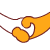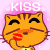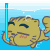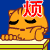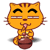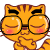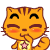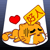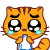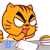12